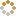﻿ N-B-U-T Online Judge ::  Encoding
• ###  Encoding

• 时间限制: 1000 ms　内存限制: 65535 K
• 问题描述
• Given a string containing only 'A' - 'Z', we could encode it using the following method:

1. Each sub-string containing k same characters should be encoded to "kX" where "X" is the only character in this sub-string.

2. If the length of the sub-string is 1, '1' should be ignored.
• 输入
• The first line contains an integer N (1 <= N <= 100) which indicates the number of test cases. The next N lines contain N strings. Each string consists of only 'A' - 'Z' and the length is less than 10000.

• 输出
• For each test case, output the encoded string in a line.
• 样例输入
• ```2
ABC
ABBCCC
```
• 样例输出
• ```ABC
A2B3C
```
• 提示
• `无`
• 来源
• `HDOJ-1020`
• 操作
﻿